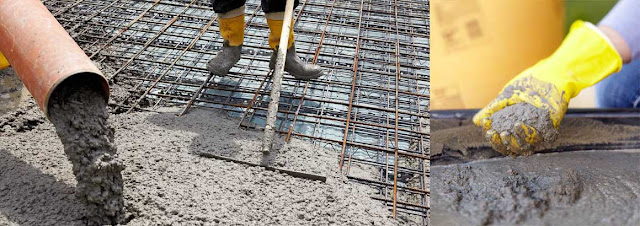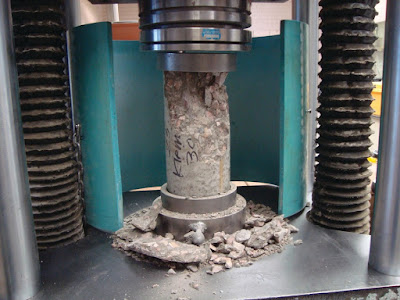## 20 Feb 2019

### Factors Affecting Concrete StrengthConcrete

## Factors Affecting Concrete Strength

In addition to Mixing, Pouring and Compaction, the concrete strength mainly depends on the following factors:Factors Affecting Concrete Strength

### (2) Aggregate-Cement Ratio

To decrease in Aggregate-Cement Ratio increase the strength of concrete upto numerical value of 2, further decrease of Agg/Cement may cause decrease in strength on concrete.

### (3) Aggregate

The concrete strength is affected by
• Aggregate Strength
• Surface Texture
• Maximum size of aggregate in concrete

### (4) Curing

Prolonged moist curing results in getting highest concrete strength.

## Rate Of Strength Gain by Concrete

The ACI code proposes the rate of strength gain for concrete in which type-I concrete was used at 70F° by the following Equation

fc'₍t ₎= fc'₍₂₈₎⎨t ⁄ 4+0.85t ⎬

In the above Equation
t= Time in days
fc'= Compressive strength at age t in days

## Variation in Strength of Concrete

The strength variation of finished concrete depends on
1. Property of concrete component
2. Methods of transportation of concrete
3. Compaction of concrete
4. Discripenses in the test of concreteCompressive Test on Concrete